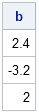The SAS/IML language provides two functions for solving a nonsingular nxn linear system A*x = c:

• The INV function numerically computes the inverse matrix, A-1. You can use this to solve for x: Ainv = inv(A); x = Ainv*c;.
• The SOLVE function numerically computes the particular solution, x, for a specific right hand side, c.

The INV function solves a general problem, whereas the SOLVE function solves a particular problem. As is often the case, solving a particular problem is easier and faster than solving a general problem. This truism applies to solving linear systems: the SOLVE function is usually faster, more efficient, and more accurate than the INV function. (See the SAS/IML documentation for details of the algorithms behind each function.)

### Generality versus Speed

Why should SOLVE be faster than INV? Here's an analogy to think about. Suppose that I ask you to solve for the intersection of two lines in the plane that are given by the equations x+y=2 and 2x-y=1. Either by graphical means or by using algebra, you easily conclude that the intersection is at the point (1,1). You've quickly solved a particular problem. (This is like calling the SOLVE function.)

Now suppose instead that I ask you to find the intersection of two lines given by the equations x+y=a and 2x-y=b, where a and b are arbitrary constants. This is a more general question, so it takes you longer to compute that the intersection is at the location ((a+b)/3, (2a-b)/3). (This is analogous to calling the INV function.) However, you now have a general answer for the location of the intersection. If I ask you for the location when a=2 and b=1, you can quickly tell me that the answer is (1,1).

After you solve the general problem, it takes very little effort to solve additional problems. (For example, you can quickly solve for the intersection when a=6 and b=3.) In addition, you gain insight into the nature of the solution. These two features indicate that solving the general problem results in several advantages. However, it comes at a cost: it takes longer to solve the general problem.

### Solving the Normal Equations

Very often, I see SAS/IML programmers use the INV function to solve the normal equations that appear in linear least squares regression.

In the least squares problem, the data are contained in a matrix x and the response values are contained in a vector y. The matrix A is the cross-product matrix x`*x and the right hand side, c, is the vector x`*y. The parameter estimates for the linear model are found by solving the linear system A*b = c for b as shown in the following SAS/IML statements:

```proc iml; /* define data; include column of 1s for intercept parameter: Int x1 x2 */ x = {1 1 1, 1 2 4, 1 3 9, 1 4 16, 1 5 25}; /* observed responses */ y = {1, 5, 9, 23, 36};   /* form normal equations */ A = x`*x; c = x`*y;   b = solve(A, c); /* solve for specific LS estimates */ print b;   Ainv = inv(A); /* solve general system */ b = Ainv * c; /* use to find LS estimates */```### Which Function Should You Use?

The SOLVE and INV functions give the same answer, but if the SOLVE function is faster, why would anyone ever use the INV function? Well, sometimes the explicit inverse is useful for computing other statistics. For example, in the least squares problem, after you compute the inverse matrix, Ainv, you can use it to estimate the covariance and standard errors of the parameter estimates. For other applications, you might want to solve the same linear system with many different right hand sides.

However, if you are interested only in a single solution, use the SOLVE function.

So the next time you solve a linear system, ask yourself, "Do I really need to compute that matrix inverse?"

ShareDistinguished Researcher in Computational Statistics

Rick Wicklin, PhD, is a distinguished researcher in computational statistics at SAS and is a principal developer of SAS/IML software. His areas of expertise include computational statistics, simulation, statistical graphics, and modern methods in statistical data analysis. Rick is author of the books Statistical Programming with SAS/IML Software and Simulating Data with SAS.

1.2.